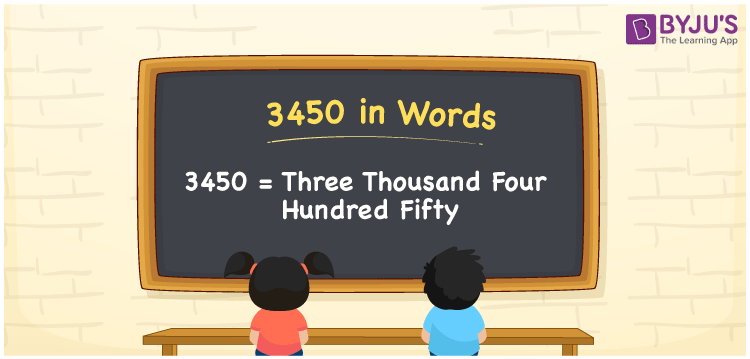# 3450 in Words

3450 in words is written as Three thousand four hundred fifty. In both the International System of Numerals and the Indian System of Numerals, 3450 is written as Three thousand four hundred fifty. The number 3450 is a Cardinal Number as it represents some quantity. For example, “that item costs 3450 rupees”.

 3450 in Words Three thousand four hundred fifty Three thousand four hundred fifty in Number 3450

## 3450 in English Words

3450 in English words is read as “Three thousand four hundred fifty”.## How to Write 3450 in Words?

To write 3450 in words, we shall use the place value chart. In the place value chart, put 3 in the thousands, 4 in the hundreds, 5 in the tens, and 0 in the ones, respectively. Let us make a place value chart to write the number 3450 in words.

 Thousands Hundreds Tens Ones 3 4 5 0

Thus, we can write the expanded form as

3 × Thousand + 4 × Hundred + 5 × Ten + 0 × One

= 3 × 1000 + 4 × 100 + 5 × 10 + 0 × 1

= 3000 + 400 + 50 + 0

= 3450

= Three thousand four hundred fifty.

3450 is a natural number which is the successor of 3449 and the predecessor of 3451.

3450 in words – Three thousand four hundred fifty

• Is 3450 an odd number? – No
• Is 3450 an even number? – Yes
• Is 3450 a perfect square number? – No
• Is 3450 a perfect cube number? – No
• Is 3450 a prime number? – No
• Is 3450 a composite number? – Yes

## Frequently Asked Questions on 3450 in Words

Q1

### How to write 3450 in words?

3450 in words is written as Three thousand four hundred fifty.
Q2

### How to write 3450 in the International and Indian System of Numerals?

In both, the system of numerals, 3450 in words, is written as Three thousand four hundred fifty.
Q3

### How to write 3450 in a place value chart?

In the place value chart, write 3 in the thousands, 4 in the hundreds, 5 in the tens, and 0 in the ones, respectively.